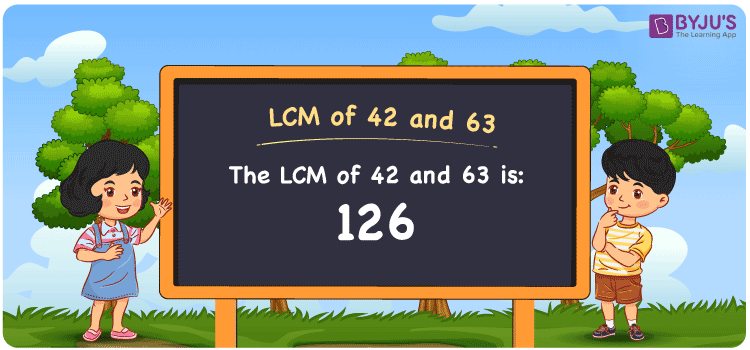Checkout JEE MAINS 2022 Question Paper Analysis : Checkout JEE MAINS 2022 Question Paper Analysis :

# LCM of 42 and 63

LCM of 42 and 63 is 126. The least common multiple of 42 and 63 can be obtained considering the common multiples. (42, 84, 126, 168, 210, ….) and (63, 126, 189, 252,….) are the multiples of 42 and 63. To find the LCM of two numbers, you can use methods such as prime factorisation, by listing the multiples and division referring to the materials provided at BYJU’S.

## What is LCM of 42 and 63?

The answer to this question is 126. The LCM of 42 and 63 using various methods is shown in this article for your reference. The LCM of two non-zero integers, 42 and 63, is the smallest positive integer 126 which is divisible by both 42 and 63 with no remainder.## How to Find LCM of 42 and 63?

LCM of 42 and 63 can be found using three methods:

• Prime Factorisation
• Division method
• Listing the multiples

### LCM of 42 and 63 Using Prime Factorisation Method

The prime factorisation of 42 and 63, respectively, is given by:

42 = 2 x 3 x 7 = 2¹ x 3¹ x 7¹

63 = 3 x 3 x 7 = 3² x 7¹

LCM (42, 63) = 126

### LCM of 42 and 63 Using Division Method

We’ll divide the numbers (42, 63) by their prime factors to get the LCM of 42 and 63 using the division method (preferably common). The LCM of 42 and 63 is calculated by multiplying these divisors.

 2 42 63 3 21 63 3 7 21 7 1 7 x 1 1

No further division can be done.

Hence, LCM (42, 63) = 126

### LCM of 42 and 63 Using Listing the Multiples

To calculate the LCM of 42 and 63 by listing out the common multiples, list the multiples as shown below

 Multiples of 42 Multiples of 63 42 63 84 126 126 189 168 252 210 315

The smallest common multiple of 42 and 63 is 126.

Therefore LCM (42, 63) = 126

## Video Lesson on Applications of LCM## LCM of 42 and 63 Solved Examples

What is the smallest number divisible exactly by 42 and 63?

Solution:

The smallest number divisible exactly by 42 and 63 is LCM.

Multiples of 42 = 42, 84, 126, 168, 210, ….

Multiples of 63 = 63, 126, 189, 252, 315, ….

Hence, the LCM is 126.

## Frequently Asked Questions on LCM of 42 and 63

### What is the LCM of 42 and 63?

The LCM of 42 and 63 is 126. To find the LCM value, we should first find the multiples of 42 and 63 and the smallest multiple divisible exactly by 42 and 63 has to be calculated.

### What methods can be used to find the LCM of 42 and 63?

The methods that can be used to find the LCM of 42 and 63 are Division Method, Listing multiples and Prime Factorization Method.

### If the LCM of 42 and 63 is 126, what is its GCF?

LCM x GCF = 42 x 63

Given

LCM of 42 and 63 = 126

126 x GCF = 2646

GCF = 2646/126 = 21